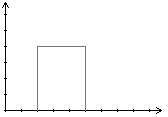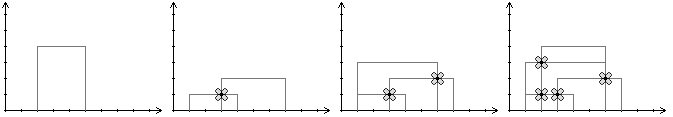### COCI 2008/2009, Croatian Regional

On a faraway planet, there are strange plants with two stems. Every plant can be described by three numbers: the x-coordinates of the stems L and R, and the height H at which the stems are connect. The image depicts a plant with L=2, R=5 and H=4.Every day a new plant grows on the planet. The plant that grows on day 1 is of height 1, and every subsequent plant is one higher than the previous one.

When a stem of a new plant intersects the horizontal segment of another plant, a small flower grows (if one wasn't there already). If segments merely touch in a point, a flower will not grow there. The following images are a visualization of the first example.Write a program that, given the coordinates of all plants, calculates the number of new flowers every day.

### Input

The first line contains an integer N (1 ≤ N ≤ 100 000), the number of days.
Each of the following N lines contains two integers L and R (1 ≤ L < R ≤ 100 000), the coordinates of the stems of a plant.

### Output

Output N lines, the number of new flowers after each plant grows.

### Sample Input

`41 43 71 62 6`

`0112`

### Sample Input

`51 33 53 92 43 8`

### Sample Output

`00032`

Point Value: 20
Time Limit: 10.00s
Memory Limit: 32M